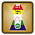Saturday, 10 November 2012

CBSE Class 9 - Science - CH3 - Atoms and Molecules (Worksheet)

Atoms and Molecules (Worksheet)Water Molecule

Fill in the blanks

1. An element has only one type of __________.

2. According to law of definite proportions, in a chemical substance the elements are always present in __________ proportions by mass.

3. According to Dalton's atomic theory, atoms of different elements differ in ______, size and chemical properties.

4. The chemical symbol of flourine is ________.

5. The chemical symbol of mercury is _________.

6. The atomicity of sulphur S8 is ________.

7. Hydrogen and oxygen combines to form H2O and H2O2. These two compounds obey the law of ____________________.

8. ____________ is formed by the loss of one or more electrons by an atom.

9. One mole of water contains ______________ molecules.

10. _________ are building block of all matter.

11. Atoms can be observed using ____________ Microscope.

12. Atomic radius is measured in __________.

13. Mass of 2 Hydrogen atoms is __________.

14. The atomic mass of  sodium is 23. The gram atomic mass of  sodium is _________.

15. A negatively charged ion is called a/an _____________.

16. The mass of  1 mole of a substance is called its _____________.

17. The _______________ of a compound is a symbolic representation of its composition.

18. ___________ is the combining capacity of an element.

19. The number of atoms present in 12 grams of carbon 12 which is equal to __________atoms . This is also known as ____________.

20. The mass of 5 moles of ammonia (NH3)  is __________.

Read study notes on Atoms and Molecules

1. atoms.
2. definite/constant
3. mass
4. F
5. Hg
6. eight (polyatomic).
7. Law of Multiple proportions
8. Ion/Cation
9. 6.023 × 1023
10. Atoms
11. Scanning Tunnelling Microscope (STM)
12. nanometres/Angstrom.
13.  2u or 2 amu
14. 23g
15. anion
16. molar mass
17. chemical formula
18. Valency
19. 6.023 × 1023 ,Avogadro's constant
20. 85g

.1.2.all questions are good but Avogadro's constant is wrong please check it out

1.instead of 6.023 it is 6.022

3.4.5.It helped alot . :)

6.Good
It is very useful

7.Good
It is very useful

8.